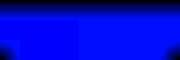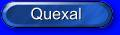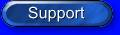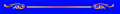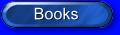#The latest trend in PC games is 3D graphics: during the past few years, almost all kinds of games have turned to 3D graphics, greatly increasing the demand of processors with strong floating-point performance, because the front end of a typical 3D game software pipeline must perform object physics, geometry transformations, clipping and lighting calculations. On the screen, 3D objects consist of thousands of polygons, usually triangles. The smaller and more numerous the polygons, the more detailed the object. When an object moves on the screen, rotates to a different viewing angle, or moves forward or backward in virtual space, the program must recalculate every vertex of every polygon. This is a geometry transformation, and it requires heavy-duty matrix multiplication: the program must multiply a 1x4 vector of coordinates against a 4x4 transform matrix. This requires up to 16 FP multiplies and 12 FP additions for each vertex, so the floating-point unit of the processor is usually the performance bottleneck of 3D games. Intels processors have always had strong numeric performance, given that they were designed not only for typical business applications, but also for scientific programs that are usually floating-point intensive. AMD processors have been designed to run business software fast, but they lack the floating-point performance of Intel chips. To gain a share of the rapidly-growing gaming market, AMD has introduced the 3D Now! instructions, designed to exceed the performance of the Intel Pentium II when running 3D games.
The AMD 3D Now! technology provides 21 additional instructions to support high-performance 3D graphics and audio processing. The 3D Now! instructions are vector instructions that operate on 64-bit registers, divided into two 32-bit single-precision floating-point words that are compatible with the IEEE-754, single-precision format. This format comprises a 1-bit sign, an 8-bit biased exponent, and a 23-bit significand with one hidden integer bit for a total of 24 bits in the significand. In contrast to the IEEE standard that dictates four rounding modes, the AMD K6 processors support only the round-to-nearest mode.
The AMD K6/Athlon processors implement eight 3D Now! registers that are mapped onto the floating-point registers just like MMX registers: aliasing the 3D Now! registers onto the floating-point stack provides a safe method to introduce this technology, because it does not require modifications to existing operating systems; furthermore it is possible to write x86 programs containing both integer, MMX and SIMD floating point instructions with no performance penalty for switching between the integer MMX and the floating-point 3D Now! units.
The following sections give a brief overview of each group of instructions in the 3D Now! set and the instructions within each group.

• Arithmetic instructions

• PFADD adds the source operand to the destination operand.
PFACC accumulates the two words of the destination operand and the source operand and stores the results in the low and high words of destination operand respectively.
PFSUB subtracts the source operand from the destination operand, while PFSUBR subtracts the destination operand from the source operand.
PFMUL multiplies the source and destination operands.
• Comparison instructions

• PFCMPEQ compares the destination operand and the source operand and generates all one bits or all zero bits based on the result of the corresponding comparison.
PFCMPGT tests if the destination operand is greater than the source operand, and PFCMPGE tests if the destination operand is greater or equal to the source operand.
PFMAX returns the larger of the two single-precision floating-point operands, PFMIN the smaller.
• Conversion instructions

• PI2FD converts a MMX register containing signed 32-bit integers to single-precision floating-point operands.
PF2ID converts a 3D Now! register containing single-precision floating-point operands to 32-bit signed integers using truncation.
• Reciprocal instructions

• PFRCP returns a low-precision estimate of the reciprocal of the source operand. Increased accuracy requires the use of two additional instructions (PFRCPIT1 and PFRCPIT2). As an example, consider the quotient  q = a/b. The PFRCP instruction quickly produces a 14-15 bit precision approximation of 1/b using an on-chip, ROM-based table lookup. A full-precision can then be computer from this approximation using the Newton-Raphson algorithm: X[i+1] =  X[i] * (2 - b*X[i]).
Given that the initial approximation X is accurate to at least 14 bits, and that the IEEE single precision contains 24 bits of mantissa, only one Newton-Raphson iteration is required. The following code sample shows the 3D Now! instructions that produce the initial approximation of the reciprocal, then compute the full-precision reciprocal from this, and lastly complete the required division:

X0 = PFRCP(b)
X1 = PFRCPIT1(b,X0)
X2 = PFRCPIT2(X1,X0)
q = PFMUL(a,X2)

The 24-bit final reciprocal value is X2. The quotient is formed in the last step by multiplying the reciprocal by the dividend a.
PFRSQRT returns a low-precision estimate of the reciprocal square root of the source operand. Increased accuracy requires the use of two additional instructions (PFRSQIT1 and PFRCPIT2).
The general Newton-Raphson reciprocal square root recurrence is:
X[i+1] = 0.5 * X[i] * (3 - b*X[i]^2)
To reduce the number of iterations, X0 is an initial approximation read from a table. The 3D Now! reciprocal square root approximation is accurate to at least 15 bits. Accordingly, to obtain a single precision 24-bit reciprocal square root of an input operand b, one Newton-Raphson iteration is required using the following 3D Now! instructions:

X0 = PFRSQRT(b)
X1 = PFMUL(X0 ,X0)
X2 = PFRSQIT1(b,X1)
X3 = PFRCPIT2(X2 ,X0)
X4 = PFMUL(b,X3)

The 24-bit final reciprocal square root value is X3.

• Integer instructions

• These operations extend the MMX instruction set. Developers should be aware that using these instructions in generic MMX code will reduce the potential market to AMD 3D Now! processors only.
PAVGUSB produces the rounded averages of the eight unsigned 8-bit integer values in the source operand and the eight corresponding unsigned 8-bit integer values in the destination operand. This instruction can be used for pixel averaging in MPEG-2 motion compensation and video scaling operations.
PMULHRW multiplies the four signed 16-bit integer values in the source operand by the four corresponding signed 16-bit integer values in the destination, then adds 8000h to the lower 16 bits of the 32-bit result, which results in the rounding of the high-order 16-bit result. The PMULHRW instruction provides a numerically more accurate result than the PMULH instruction, which truncates the result instead of rounding.
• Other instructions

• In applications where a large number of data sets must be processed, the PREFETCH instruction can pre-load the next data set into the data cache while the processor is simultaneously operating on the present set of data. When the present set of data values is completed, the next set is already available in the data cache, greatly reducing the delays due to cache misses. An example of a concurrent operation is vertices processing in 3D transformations, where the next set of vertices can be prefetched into the data cache while the present set is being transformed.
FEMMS offers a faster context switch at the end of an MMX routine than EMMS.

Update: there are rumours that the next Athlon core, code-named Palomino, will support SSE instructions. Current AMD processors already support integer SSE instructions, but the adoption of floating-point SSE instructions means the end of 3D-Now!. AMD's market share is steadily rising, due to its excellent price / performance ratio, but it still does not grab more than one quarter of the market; this means that developer are more likely to write SSE code than 3D-Now! code. At the same time, SSE is a more powerful instruction set, designed without the compatibility problems that limited the level of parallelism of 3D-Now!.

Update: the Athlon XP processor supports SSE instructions (curiously named 3DNow! Pro)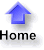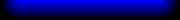Copyright Stefano Tommesani 2000/03 - All trademarks belong to their respective holders